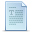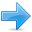# Friendship and inheritance

### Friend functions

In principle, private and protected members of a class cannot be accessed from outside the same class in which they are declared. However, this rule does not apply to "friends".

Friends are functions or classes declared with the `friend` keyword.

A non-member function can access the private and protected members of a class if it is declared a friend of that class. That is done by including a declaration of this external function within the class, and preceding it with the keyword `friend`:

 ``12345678910111213141516171819202122232425262728`` ``````// friend functions #include using namespace std; class Rectangle { int width, height; public: Rectangle() {} Rectangle (int x, int y) : width(x), height(y) {} int area() {return width * height;} friend Rectangle duplicate (const Rectangle&); }; Rectangle duplicate (const Rectangle& param) { Rectangle res; res.width = param.width*2; res.height = param.height*2; return res; } int main () { Rectangle foo; Rectangle bar (2,3); foo = duplicate (bar); cout << foo.area() << '\n'; return 0; }`````` `24`

The `duplicate` function is a friend of class `Rectangle`. Therefore, function `duplicate` is able to access the members `width` and `height` (which are private) of different objects of type `Rectangle`. Notice though that neither in the declaration of `duplicate` nor in its later use in `main`, function `duplicate` is considered a member of class `Rectangle`. It isn't! It simply has access to its private and protected members without being a member.

Typical use cases of friend functions are operations that are conducted between two different classes accessing private or protected members of both.

### Friend classes

Similar to friend functions, a friend class is a class whose members have access to the private or protected members of another class:

 ``12345678910111213141516171819202122232425262728293031323334`` ``````// friend class #include using namespace std; class Square; class Rectangle { int width, height; public: int area () {return (width * height);} void convert (Square a); }; class Square { friend class Rectangle; private: int side; public: Square (int a) : side(a) {} }; void Rectangle::convert (Square a) { width = a.side; height = a.side; } int main () { Rectangle rect; Square sqr (4); rect.convert(sqr); cout << rect.area(); return 0; }`````` `16`

In this example, class `Rectangle` is a friend of class `Square` allowing `Rectangle`'s member functions to access private and protected members of `Square`. More concretely, `Rectangle` accesses the member variable `Square::side`, which describes the side of the square.

There is something else new in this example: at the beginning of the program, there is an empty declaration of class `Square`. This is necessary because class `Rectangle` uses `Square` (as a parameter in member `convert`), and `Square` uses `Rectangle` (declaring it a friend).

Friendships are never corresponded unless specified: In our example, `Rectangle` is considered a friend class by `Square`, but Square is not considered a friend by `Rectangle`. Therefore, the member functions of `Rectangle` can access the protected and private members of `Square` but not the other way around. Of course, `Square` could also be declared friend of `Rectangle`, if needed, granting such an access.

Another property of friendships is that they are not transitive: The friend of a friend is not considered a friend unless explicitly specified.

### Inheritance between classes

Classes in C++ can be extended, creating new classes which retain characteristics of the base class. This process, known as inheritance, involves a base class and a derived class: The derived class inherits the members of the base class, on top of which it can add its own members.

For example, let's imagine a series of classes to describe two kinds of polygons: rectangles and triangles. These two polygons have certain common properties, such as the values needed to calculate their areas: they both can be described simply with a height and a width (or base).

This could be represented in the world of classes with a class `Polygon` from which we would derive the two other ones: `Rectangle` and `Triangle`:The `Polygon` class would contain members that are common for both types of polygon. In our case: `width` and `height`. And `Rectangle` and `Triangle` would be its derived classes, with specific features that are different from one type of polygon to the other.

Classes that are derived from others inherit all the accessible members of the base class. That means that if a base class includes a member `A` and we derive a class from it with another member called `B`, the derived class will contain both member `A` and member `B`.

The inheritance relationship of two classes is declared in the derived class. Derived classes definitions use the following syntax:

``` class derived_class_name: public base_class_name { /*...*/ }; ```
Where `derived_class_name` is the name of the derived class and `base_class_name` is the name of the class on which it is based. The `public` access specifier may be replaced by any one of the other access specifiers (`protected` or `private`). This access specifier limits the most accessible level for the members inherited from the base class: The members with a more accessible level are inherited with this level instead, while the members with an equal or more restrictive access level keep their restrictive level in the derived class.

 ``````// derived classes #include using namespace std; class Polygon { protected: int width, height; public: void set_values (int a, int b) { width=a; height=b;} }; class Rectangle: public Polygon { public: int area () { return width * height; } }; class Triangle: public Polygon { public: int area () { return width * height / 2; } }; int main () { Rectangle rect; Triangle trgl; rect.set_values (4,5); trgl.set_values (4,5); cout << rect.area() << '\n'; cout << trgl.area() << '\n'; return 0; }`````` ```20 10```

The objects of the classes `Rectangle` and `Triangle` each contain members inherited from `Polygon`. These are: `width`, `height` and `set_values`.

The `protected` access specifier used in class `Polygon` is similar to `private`. Its only difference occurs in fact with inheritance: When a class inherits another one, the members of the derived class can access the protected members inherited from the base class, but not its private members.

By declaring `width` and `height` as `protected` instead of `private`, these members are also accessible from the derived classes `Rectangle` and `Triangle`, instead of just from members of `Polygon`. If they were public, they could be accessed just from anywhere.

We can summarize the different access types according to which functions can access them in the following way:

Access`public``protected``private`
members of the same classyesyesyes
members of derived classyesyesno
not membersyesnono

Where "not members" represents any access from outside the class, such as from `main`, from another class or from a function.

In the example above, the members inherited by `Rectangle` and `Triangle` have the same access permissions as they had in their base class `Polygon`:

 ``12345`` ``````Polygon::width // protected access Rectangle::width // protected access Polygon::set_values() // public access Rectangle::set_values() // public access ``````

This is because the inheritance relation has been declared using the `public` keyword on each of the derived classes:

 ``1`` ``class Rectangle: public Polygon { /* ... */ }``

This `public` keyword after the colon (`:`) denotes the most accessible level the members inherited from the class that follows it (in this case `Polygon`) will have from the derived class (in this case `Rectangle`). Since `public` is the most accessible level, by specifying this keyword the derived class will inherit all the members with the same levels they had in the base class.

With `protected`, all public members of the base class are inherited as `protected` in the derived class. Conversely, if the most restricting access level is specified (`private`), all the base class members are inherited as `private`.

For example, if daughter were a class derived from mother that we defined as:

 ``1`` ``class Daughter: protected Mother;``

This would set `protected` as the less restrictive access level for the members of `Daughter` that it inherited from mother. That is, all members that were `public` in `Mother` would become `protected` in `Daughter`. Of course, this would not restrict `Daughter` from declaring its own public members. That less restrictive access level is only set for the members inherited from `Mother`.

If no access level is specified for the inheritance, the compiler assumes private for classes declared with keyword `class` and public for those declared with `struct`.

Actually, most use cases of inheritance in C++ should use public inheritance. When other access levels are needed for base classes, they can usually be better represented as member variables instead.

### What is inherited from the base class?

In principle, a publicly derived class inherits access to every member of a base class except:

• its constructors and its destructor
• its assignment operator members (operator=)
• its friends
• its private members

Even though access to the constructors and destructor of the base class is not inherited as such, they are automatically called by the constructors and destructor of the derived class.

Unless otherwise specified, the constructors of a derived class calls the default constructor of its base classes (i.e., the constructor taking no arguments). Calling a different constructor of a base class is possible, using the same syntax used to initialize member variables in the initialization list:

``` derived_constructor_name (parameters) : base_constructor_name (parameters) {...} ```
For example:

 ``````// constructors and derived classes #include using namespace std; class Mother { public: Mother () { cout << "Mother: no parameters\n"; } Mother (int a) { cout << "Mother: int parameter\n"; } }; class Daughter : public Mother { public: Daughter (int a) { cout << "Daughter: int parameter\n\n"; } }; class Son : public Mother { public: Son (int a) : Mother (a) { cout << "Son: int parameter\n\n"; } }; int main () { Daughter kelly(0); Son bud(0); return 0; }`````` ```Mother: no parameters Daughter: int parameter Mother: int parameter Son: int parameter```

Notice the difference between which `Mother`'s constructor is called when a new `Daughter` object is created and which when it is a `Son` object. The difference is due to the different constructor declarations of `Daughter` and `Son`:

 ``12`` ``````Daughter (int a) // nothing specified: call default constructor Son (int a) : Mother (a) // constructor specified: call this specific constructor ``````

### Multiple inheritance

A class may inherit from more than one class by simply specifying more base classes, separated by commas, in the list of a class's base classes (i.e., after the colon). For example, if the program had a specific class to print on screen called `Output`, and we wanted our classes `Rectangle` and `Triangle` to also inherit its members in addition to those of `Polygon` we could write:

 ``12`` ``````class Rectangle: public Polygon, public Output; class Triangle: public Polygon, public Output;``````

Here is the complete example:

 ``````// multiple inheritance #include using namespace std; class Polygon { protected: int width, height; public: Polygon (int a, int b) : width(a), height(b) {} }; class Output { public: static void print (int i); }; void Output::print (int i) { cout << i << '\n'; } class Rectangle: public Polygon, public Output { public: Rectangle (int a, int b) : Polygon(a,b) {} int area () { return width*height; } }; class Triangle: public Polygon, public Output { public: Triangle (int a, int b) : Polygon(a,b) {} int area () { return width*height/2; } }; int main () { Rectangle rect (4,5); Triangle trgl (4,5); rect.print (rect.area()); Triangle::print (trgl.area()); return 0; }`````` ```20 10 ```Previous:Special membersIndexNext:Polymorphism